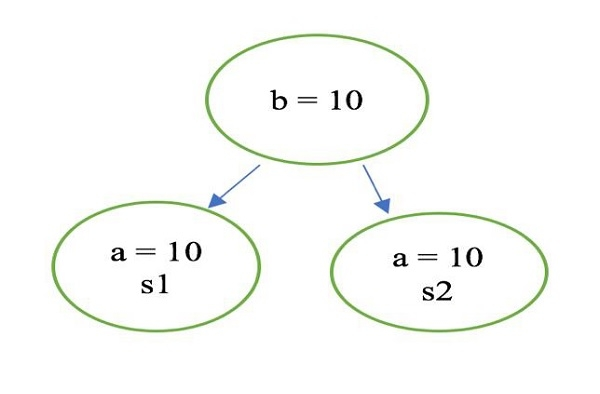# Where and how to use static variable in swift?

Before we see where and how to use static variable, let us first understand, What is static variable in swift?

## Static Variable

Static variables are those variables whose values are shared among all the instance or object of a class. When we define any variable as static, it gets attached to a class rather than an object. The memory for the static variable will be allocation during the class loading time.Let us understand above figure, we have a class Sample and it has two object s1 and s2. You see s1 and s2 both have their variable “a” separately but they have common shared variable “b”. This “b” is static variable.

Now let us see where and how we should be using static variables.

You create static variable by appending static keyword in front of your variable declaration.

static let/var variable name: type(optional) = value
static let num1: Int = 1
static var name1 = “Akash”

We will be using playground to explore more.

Open Xcode → File → Playground and name it “staticvariables”

When we define any variable as let, it means it’s values cannot be modified, On the other hand if we define any variable as var it means it’s values can be modified.

class Student {
static let section: String = "A"  // static constat
static var day: String = "Monday" // static variable
var name: String = "Akash"        // instance variable
var rollNum: Int = 1              // instance variable
}
let student1 = Student()   // Object 1
print(student1.name)       // Akash
print(student1.rollNum )   // 1
student1.name = "Aman"     // Setting ob1 value to Aman
print(student1.name) // Aman
let student2 = Student() // Object 2
print(student2.name) // Akash
print(Student.section) // A
print(Student.day) // Monday